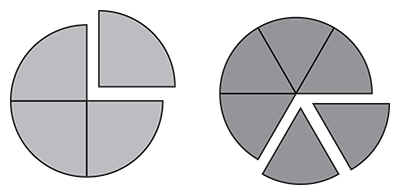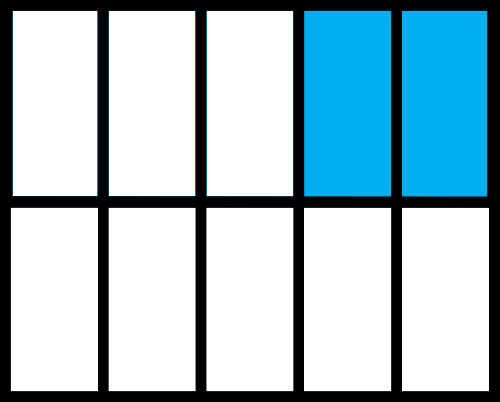Representing Fractions
Equivalent Fractions
Multiplying Fractions
Making Fraction
Find one Equivalent Fraction
100

What fraction does this picture represents?2/4

100

1/2 = ? /10

5

100

2/4 x 4/4 =

8/16

100

Find the fraction:

A cube has 6 square faces. One of them is shaded. Represent the shaded part in a fraction?

1/6

100

2/3=

100

Example; 4/8 + 1/8 = 5/8

Find 3/6+2/6

5/6

200

Which fraction is shown below?D) 1/2

200

3/4 = 12/?

16

200

2/6 x 6/6 =

8/36

200

A cube has 6 square faces. One of them is shaded. Represent the not shaded parts of the cube using a fraction form.

5/6

200

10/20=

200

Example; 4/8 + 1/8 = 5/8

6/7 + 5/7

11/7

300

State the two fractions represented below.First image 1/4

Second image 2/6

300

7/8 = 14/?

16

300

5/8 + 3/3 =

15/24

300

In a box of 12 crayons, 4 are red color, the rest assorted. Write the fraction of red crayons in this box.

4/12

300

5/7=

300

Example; 4/8 + 1/8 = 5/8

1/8 + 3/8 =4/8

4/8

400

Which fraction is represented below?D) 3/8

400

18/20 = 9/?

10

400

3/2 + 6/6 =

18/12

400

In a box of 12 crayons, 4 are red color, the rest assorted. Write the fraction assorted crayons in this box.

8/12

400

50/120=

400

Example; 4/8 + 1/8 = 5/8

2/5 +3/5 =

5/5 or 1 whole

500

Identify the fraction below?D) 2/10

500

35/80 = ?/16

7

500

Val's construction team was supposed to build a frame 4/10 meters long, but they ended up building the frame 12/30 long. How long was the frame Val's team built? Was the frame build as supposed by the team?

Val's team was built as previously supposed because 4/10=12/30

500

Ruth needs 3/4 cups of flour for one cake. She needs  the same amount of cups of flour for another cake, but for the last cake she needs to divide the flour into 8 equal part. Write the fraction of Ruth's second cake' flour.

500

36/70=